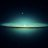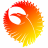# Normalized MACD Example Study for ThinkorSwim

T

#### tomsk

##### Well-known member
VIP
Warehouse
Folks

There was some discussion in one of the sub forums regarding how to normalize plots.
Rather than post it there, thought it might make it easier for future searches to find it here

Based on a normalizePlot function Mobius wrote several years ago, you can use that to normalize plots to whatever scale you like, e.g. 0 to 100, -100 to 100, etc
Here is an example on how to normalize the MACD on a scale from -100 to 100.

First determine what data you're going to plot. In the example below I have chosen to plot the MACDAvg line
Place all the code into a script() routine, in my example I call this MACDScr()
Then run this through Mobius normalizePlot function and slap on whatever lipstick you need

Code:
``````# Normalized MACD
# tomsk
# 11.16.2019

declare lower;

input fastLength = 12;
input slowLength = 26;
input MACDLength = 9;
input OverBought = 80;
input OverSold = -80;

script normalizePlot {
input data = close;
input newRngMin =  -1;
input newRngMax = 1;
def hhData = HighestAll( data );
def llData = LowestAll( data );
plot nr = ((( newRngMax - newRngMin ) * ( data - llData )) / ( hhData - llData )) + newRngMin;
}

script MACDScr {
input fastLength = 13;
input slowLength = 21;
input MACDLength  =  8;
input data = close;
def Value = ExpAverage(data, fastLength) - ExpAverage(data, slowLength);
plot MACDAvg  = ExpAverage(Value, MACDLength);
}

plot MACDX = normalizePlot(MACDScr("fastLength" = fastLength, "slowLength" = slowLength, "MACDLength" = MACDLength, "data" = close), -100, 100);

plot OS = if !isNaN(close) then OverSold else Double.NaN;
OS.SetPaintingStrategy(PaintingStrategy.Line);
OS.SetLineWeight(2);
OS.SetDefaultColor(Color.Cyan);

plot OB = if !IsNaN(close) then OverBought else Double.NaN;
OB.SetPaintingStrategy(PaintingStrategy.Line);
OB.SetLineWeight(2);
OB.SetDefaultColor(Color.Cyan);

plot MID = if !isNaN(close) then 0 else Double.NaN;
MID.SetPaintingStrategy(PaintingStrategy.Dashes);
MID.SetLineWeight(2);
MID.SetDefaultColor(Color.Orange);

MACDX.SetPaintingStrategy(PaintingStrategy.Line);
MACDX.SetLineWeight(1);
MACDX.AssignValueColor(if MACDX >= 50 then if MACDX > MACDX then Color.Light_Green else Color.Light_Red else if MACDX < MACDX then Color.Light_Red else Color.Light_Green);Indicators 3Indicators 7